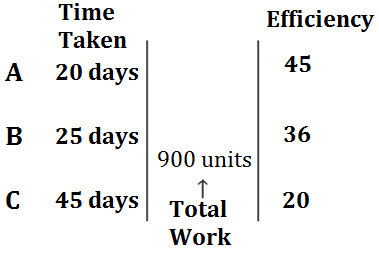Question of The Day20-07-2020

Three persons A, B and C can complete a project in 20, 25 and 45 days respectively. All of them decided to work together, but after 3 days A left the group and C left the group 2 days before the completion of the work. For how many days B did the work on the project?

Correct Answer : a ) $$14 {3 \over 8}days$$

Explanation :

Quick Approach

Let us assume total work be LCM (20, 25 and 45 days) = 900 unitsFrom the above figure we can say that

Work done in the first 3 days = (Efficiency of A, B, and C) * 3 = (45 + 36 + 20) * 3 = 101 * 3 = 303 units

Now, as in the last 2 days C also left the group so in last 2 days work will be done by only B

Thus, work done in last 2 days = 2 * efficiency of B = 2 * 36 = 72 units

Now, remaining work = 900 – (303 + 72) = 525 units

As, the remaining work was complete by both B and C so,

Time taken by B and C to complete the work=$${525 \over (36+20)}={525 \over 56}=9{3 \over 8} \space days$$

Now, time for which B worked on the project will be the total time in which the whole project will be completed

So, total time to finish the work will be

$$3+ 9{3 \over 8}+2=14 { 3 \over 8} \space days$$

Hence, (a) is the correct answer.

Basic Approach

Let us assume total work be completed in X days

A, B, and C alone can complete a piece of work in 20, 25 and 45 days respectively

1 day work of A=$${1 \over 20}$$

1 day work of B=$${1 \over 25}$$

1 day work of C=$${1 \over 45}$$

Therefore,

Work completed by A, B, and C together in 1 day

=$${1 \over 20}+{1 \over 25}+{1 \over 45} ={101 \over 900}$$

Work completed by A, B, and C together in 3 days=$$3* {101 \over 900}$$=$${303 \over 900}$$

Now, 1 day work of B and C together=$${1 \over 25}+{1 \over 45}={9+5 \over 225}={14 \over 225}$$

According to the question,

$${303 \over 900}+(x-5){14 \over 225}+{2 \over 25}=1$$

$${303+56(x-5)+72 \over 900}=1$$

$${303+56x-280+72 \over 900}=1$$

$$x={805 \over 56}=14 {3 \over 8} \space days$$

Hence, (a) is the correct answer.

Such type of question is asked in various government exams like SSC CGL, SSC MTS, SSC CPO, SSC CHSL, RRB JE, RRB NTPC, RRB GROUP D, RRB OFFICER SCALE-I, IBPS PO, IBPS SO, RRB Office Assistant, IBPS Clerk, RBI Assistant, IBPS RRB OFFICER SCALE 2&3, UPSC CDS, UPSC NDA etc.

Read Daily Current Affairs, Banking Awareness, Hindi Current Affairs, Word of the Day, and attempt free mock tests at PendulumEdu and boost your preparation for the actual exam.0